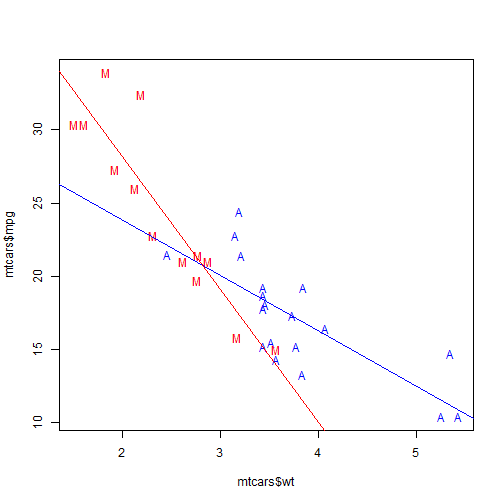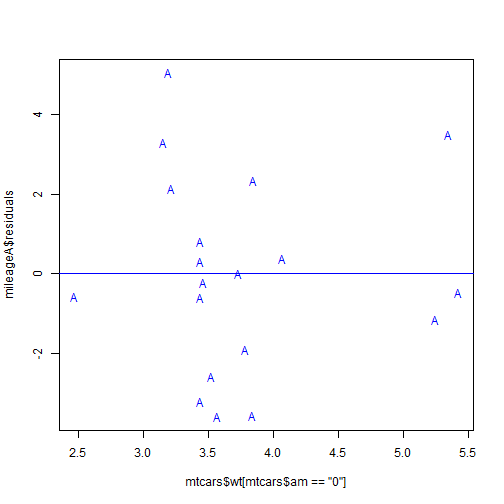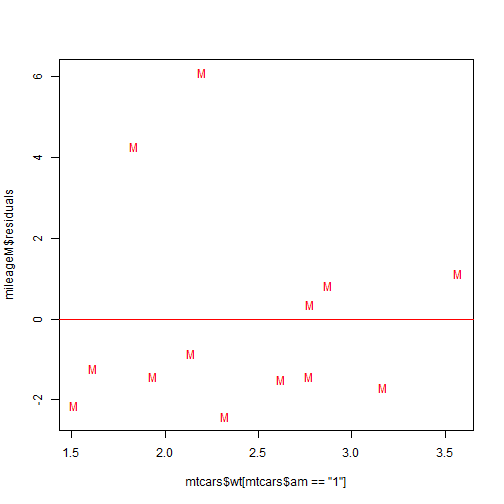# Regression Models

## Executive Summary

Pretend we work for Motor Trend, a magazine about the automobile industry. Looking at a data set of a collection of cars, we are interested in exploring the relationship between a set of variables and miles per gallon (MPG). Let us focus on answering the following two questions:

1. “Is an automatic or manual transmission better for MPG?”
2. “How different is the MPG between automatic and manual transmissions?”

## Exploratory Data Analysis

We will use the data presented by Henderson and Velleman in their 1981 article “Building Multiple Regression Models Interactively” from Biometrics. The R program includes this dataset for practice, and we can load that dataset with

``````library(UsingR)
``````
``````## Loading required package: MASS
``````
``````data(mtcars)
``````
``````##                    mpg cyl disp  hp drat    wt  qsec vs am gear carb
## Mazda RX4         21.0   6  160 110 3.90 2.620 16.46  0  1    4    4
## Mazda RX4 Wag     21.0   6  160 110 3.90 2.875 17.02  0  1    4    4
## Datsun 710        22.8   4  108  93 3.85 2.320 18.61  1  1    4    1
## Hornet 4 Drive    21.4   6  258 110 3.08 3.215 19.44  1  0    3    1
## Hornet Sportabout 18.7   8  360 175 3.15 3.440 17.02  0  0    3    2
## Valiant           18.1   6  225 105 2.76 3.460 20.22  1  0    3    1
``````

The column am classifies the cars' transmissions. We can focus on the MPG results for the automatic and manual transmissions respectively with

``````summary(mtcars\$mpg[mtcars\$am == "0"])
``````
``````##    Min. 1st Qu.  Median    Mean 3rd Qu.    Max.
##    10.4    15.0    17.3    17.1    19.2    24.4
``````
``````summary(mtcars\$mpg[mtcars\$am == "1"])
``````
``````##    Min. 1st Qu.  Median    Mean 3rd Qu.    Max.
##    15.0    21.0    22.8    24.4    30.4    33.9
``````

Across all of the indicated quantiles and the means, we infer that the cars with manual transmissions acheive better gas mileage. In particular, the difference in the means is

``````mean(mtcars\$mpg[mtcars\$am == "1"]) - mean(mtcars\$mpg[mtcars\$am == "0"])
``````
``````##  7.245
``````

## Regression Models

To be more thorough in our approach, let us now apply linear regression models comparing the cars' gas mileage to their weights (so that a consumer can also decide on the size of the car)—accounting for the transmission types:

``````mileageA <- lm(mtcars\$mpg[mtcars\$am == "0"] ~ mtcars\$wt[mtcars\$am == "0"])
mileageM <- lm(mtcars\$mpg[mtcars\$am == "1"] ~ mtcars\$wt[mtcars\$am == "1"])
``````

This time, when we look coefficients of those models,

``````summary(mileageA)\$coefficients
``````
``````##                             Estimate Std. Error t value  Pr(>|t|)
## (Intercept)                   31.416     2.9467  10.661 6.008e-09
## mtcars\$wt[mtcars\$am == "0"]   -3.786     0.7666  -4.939 1.246e-04
``````
``````summary(mileageM)\$coefficients
``````
``````##                             Estimate Std. Error t value  Pr(>|t|)
## (Intercept)                   46.294      3.120  14.839 1.277e-08
## mtcars\$wt[mtcars\$am == "1"]   -9.084      1.257  -7.229 1.688e-05
``````

we can see that the cars with automatic transmission lose about 3.8 MPG per 1000 pounds in weight, while the cars with manual transmissions lose about 9.1 MPG per 1000 pounds in weight. There might be an intersection point where—at a certain weight—both types of transmissions tend to yield virtually equal gas mileage. However, would that intersection take place at a point for real-world cars? (The image is in the appendices)

From here, we can investigate the residuals from each linear regression model. The means and covariances are

``````mean(mileageA\$residuals)
``````
``````##  -2.163e-16
``````
``````mean(mileageM\$residuals)
``````
``````##  8.537e-17
``````
``````cov(mileageA\$residuals, mtcars\$wt[mtcars\$am == "0"])
``````
``````##  5.001e-16
``````
``````cov(mileageM\$residuals, mtcars\$wt[mtcars\$am == "1"])
``````
``````##  2.198e-16
``````

In each of those 4 calculations, we get virtually zero (as desired).

## Appendices

Let us now look at the visuals for our linear regression models. I will label the automatic transmission cars with blue “A” symbols, and manual transmission cars with red “M” symbols.

``````plot(mtcars\$wt, mtcars\$mpg, pch = 21, col = "white")
points(mtcars\$wt[mtcars\$am == "0"], mtcars\$mpg[mtcars\$am == "0"], pch = "A",
col = "blue")
points(mtcars\$wt[mtcars\$am == "1"], mtcars\$mpg[mtcars\$am == "1"], pch = "M",
col = "red")
abline(mileageA, col = "blue")
abline(mileageM, col = "red")
``````We see some curious patterns. First, it appears that the manual transmission cars tend to be lighter than the automatic transmission cars. Furthermore, our linear regression lines (with the same colors:red for manual transmissions, blue for automatic transmissions) do intersect within the dataset, so perhaps automatic transmissions are the better choice for heavier cars.

Finally, we can look at the residuals from the linear regression models for the automatic and the manual transmission cars respectively. We see once again how the residuals “cancel” out.

``````plot(mtcars\$wt[mtcars\$am == "0"], mileageA\$residuals, pch = "A", col = "blue")
abline(lm(mileageA\$residuals ~ mtcars\$wt[mtcars\$am == "0"]), col = "blue")
````````````plot(mtcars\$wt[mtcars\$am == "1"], mileageM\$residuals, pch = "M", col = "red")
abline(lm(mileageM\$residuals ~ mtcars\$wt[mtcars\$am == "1"]), col = "red")
``````We could probably do further analysis by breaking down the dataset into factors based on the number of cylinders in the engines (4,6,8). However, the dataset intially had only 32 observations, so further analysis with small sample sizes might not yield desired P-values.